﻿ 一起聊聊Excel的SUMPRODUCT函数 - 自由资讯

# 一起聊聊Excel的SUMPRODUCT函数## 基础语法

`=SUMPRODUCT（array1,array2,array3, …）`

——SUM是求和的意思，PRODUCT是相乘的意思，参数之间相乘之后再求和，你看，SUMPRODUCT确实人如其名了。

1> 它本身默认执行数组运算。

2> 它会将参数中非数值型的数组元素作为0处理。

3> 参数必须有相同的尺寸，否则返回错误值。

## 特点解析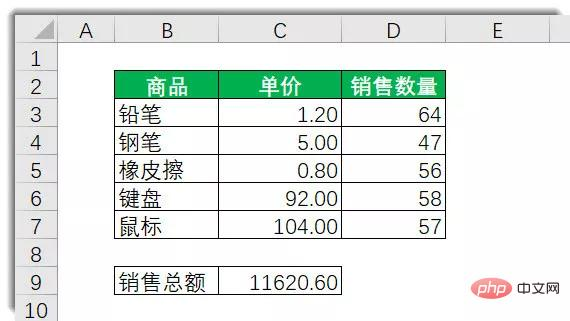C9输入以下公式，即可得出结果11620.60

`=SUMPRODUCT(C3:C7, D3:D7)`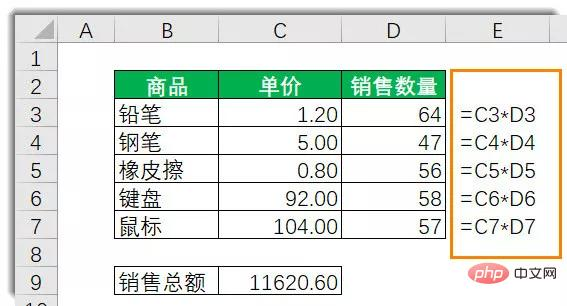`=SUMPRODUCT(C3:C7*D3:D7)`

`=SUM(C3:C7*D3:D7)`

……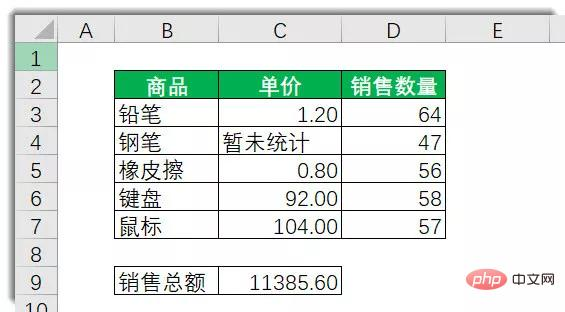`=SUMPRODUCT(C3:C7*D3:D7)`

`=SUM(C3:C7*D3:D7)`

=SUMPRODUCT(C3:C7,D3:D7)

……

`=SUMPRODUCT(C3:C7,D3:D6)`

——于是SUMPRODUCT就不高兴了，它给你一个错误值#VALUE!，明确告诉你，和谐时代幸福岁月，日子不能这么过。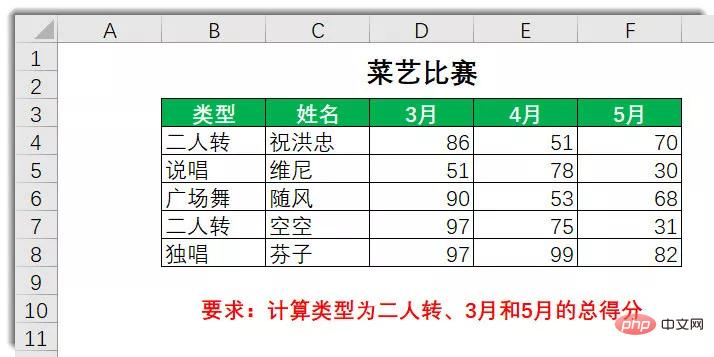## 案例拓展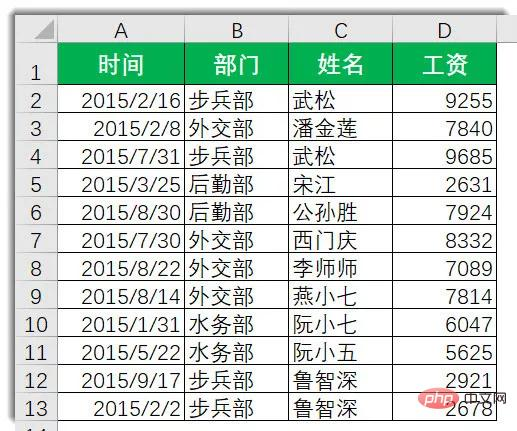——那么，问题和广告都来了：

1

`=SUMPRODUCT((C2:C13="西门庆")*1)`

2

`=SUMPRODUCT((C2:C13="西门庆")*D2:D13)`

3

`=SUMPRODUCT((MONTH(A2:A13)=2)*(B2:B13="外交部"))`

……

SUMPRODUCT跃然而至：

`=SUMPRODUCT((MONTH(A2:A13)=6)*(B2:B13="外交部"),D2:D13)`

`=SUMPRODUCT((MONTH(A2:A13)=6)*(B2:B13="外交部")*D2:D13)`

`=SUMPRODUCT((条件一)*(条件二)……,求和区域)`

4

`=SUMPRODUCT((MONTH(A2:A13)=2)*(B2:B13="外交部")*D2:D13)+SUMPRODUCT((MONTH(A2:A13)=2)*(B2:B13="步兵部")*D2:D13)`

`=SUMPRODUCT((MONTH(A2:A13)=6)*(B2:B13={"外交部","步兵部"})*D2:D13)`

5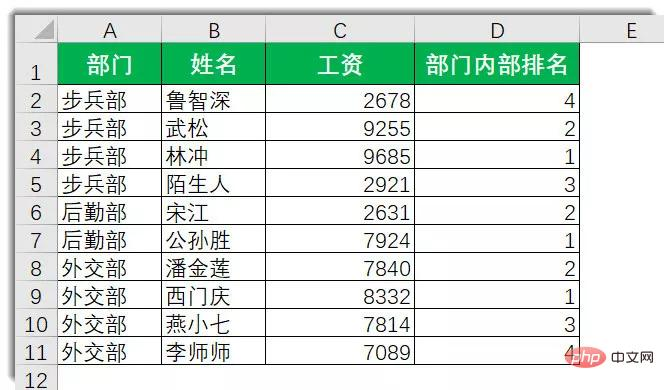SUMPRODUCT是这么解决这个问题的，D2输入公式向下复制：

`=SUMPRODUCT((\$A\$2:\$A\$9=A2)*(C2<\$C\$2:\$C\$9))+1`

（思考，为什么公式的最后+1，而不是直接写成如下：）

`=SUMPRODUCT((\$A\$2:\$A\$9=A2)*(C2<=\$C\$2:\$C\$9))`

## 结束语

`=SUMPRODUCT((MONTH(A2:A13)=6)*(B2:B13="财务部")*D2:D13)`

`=SUMPRODUCT((MONTH(A2:A13)=6)*(B2:B13="财务部")，D2:D13)`

SUMPRODUCT为什么有时候比SUMIF/COUNTIF计算速度慢？【本文转自：海外高防服务器 http://www.558idc.com/krgf.html 欢迎留下您的宝贵建议】

﻿